Ex 12.1

Chapter 12 Class 6 Ratio and Proportion
Serial order wise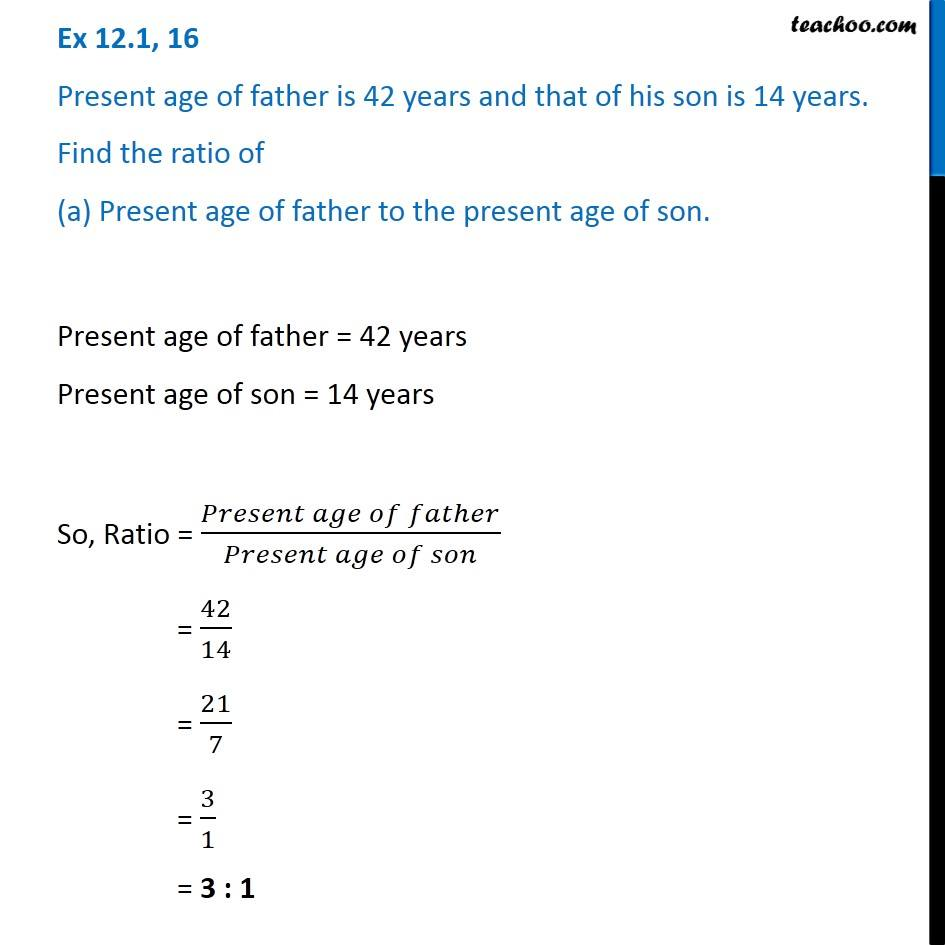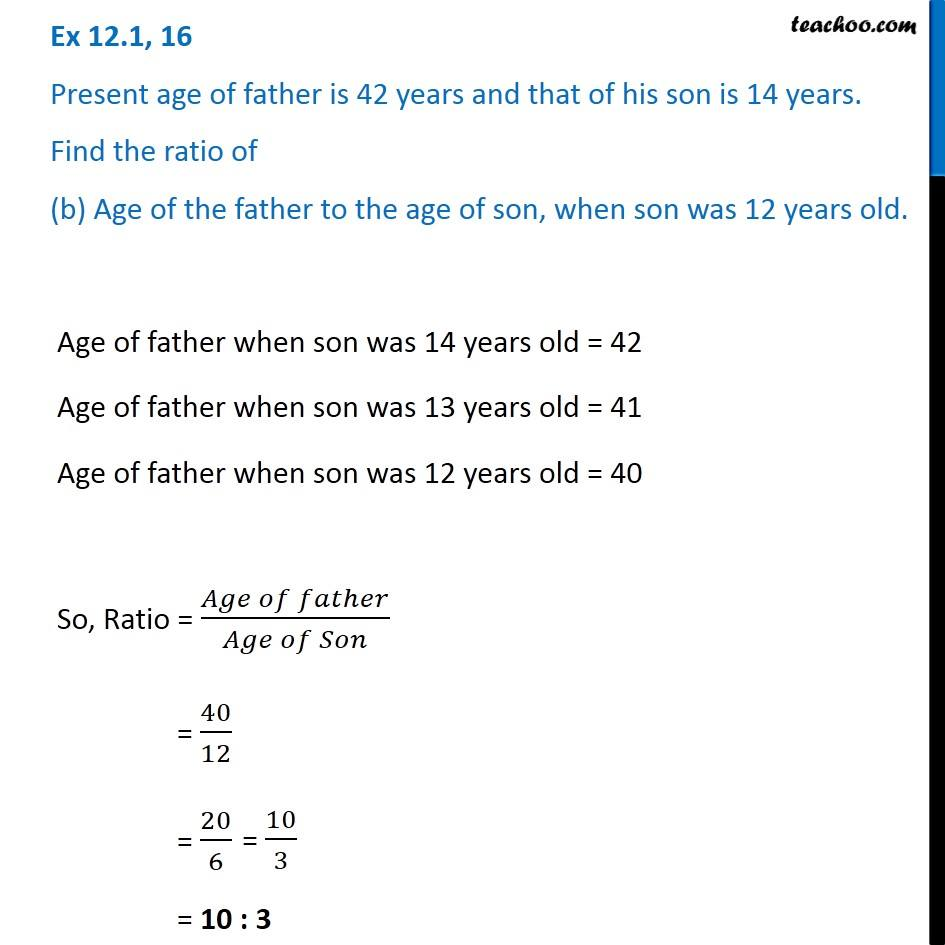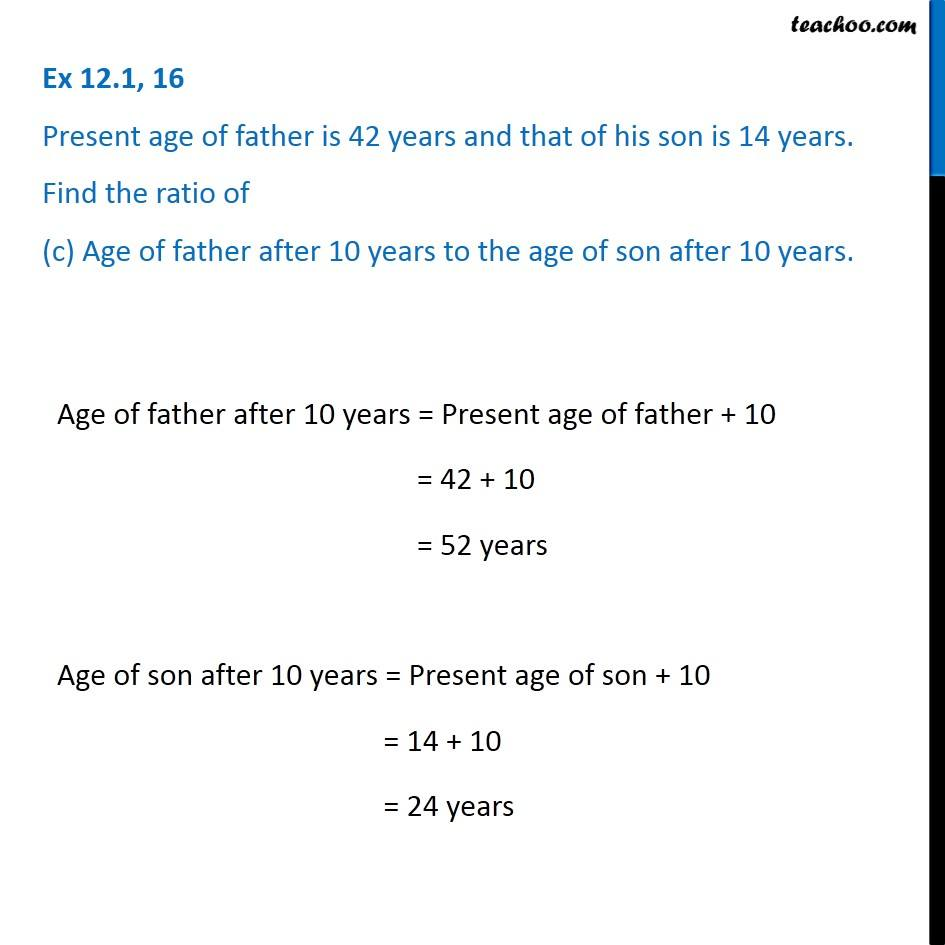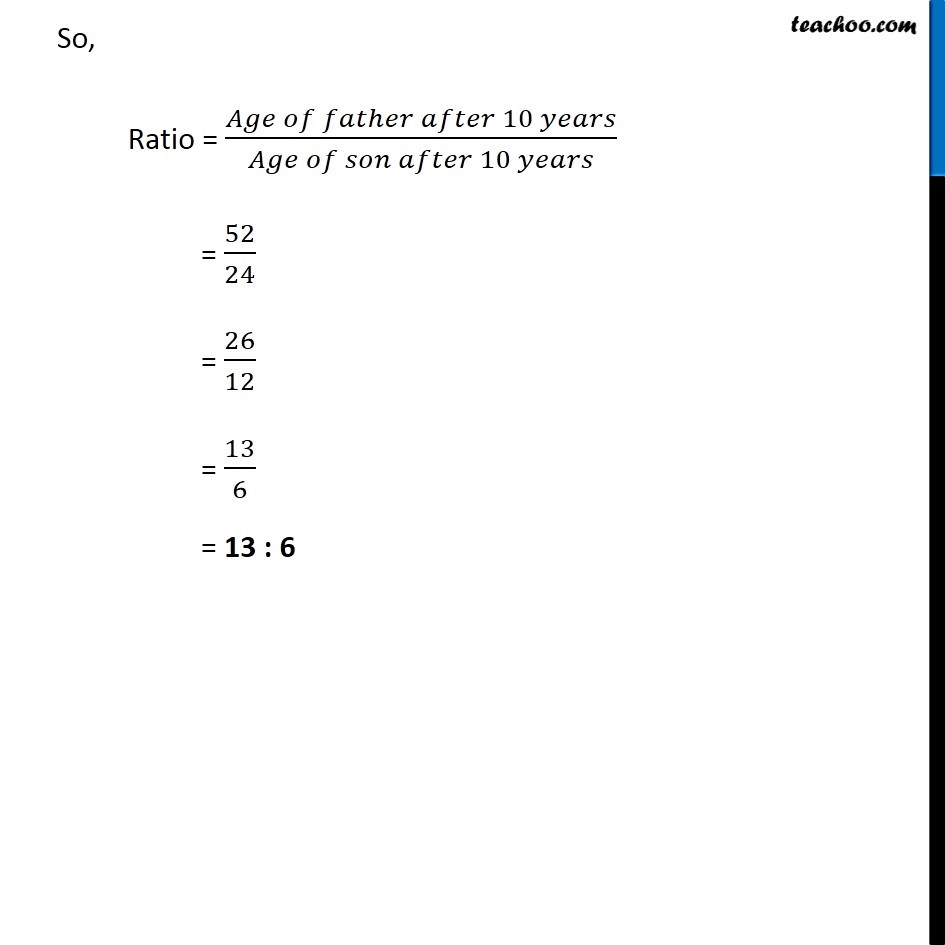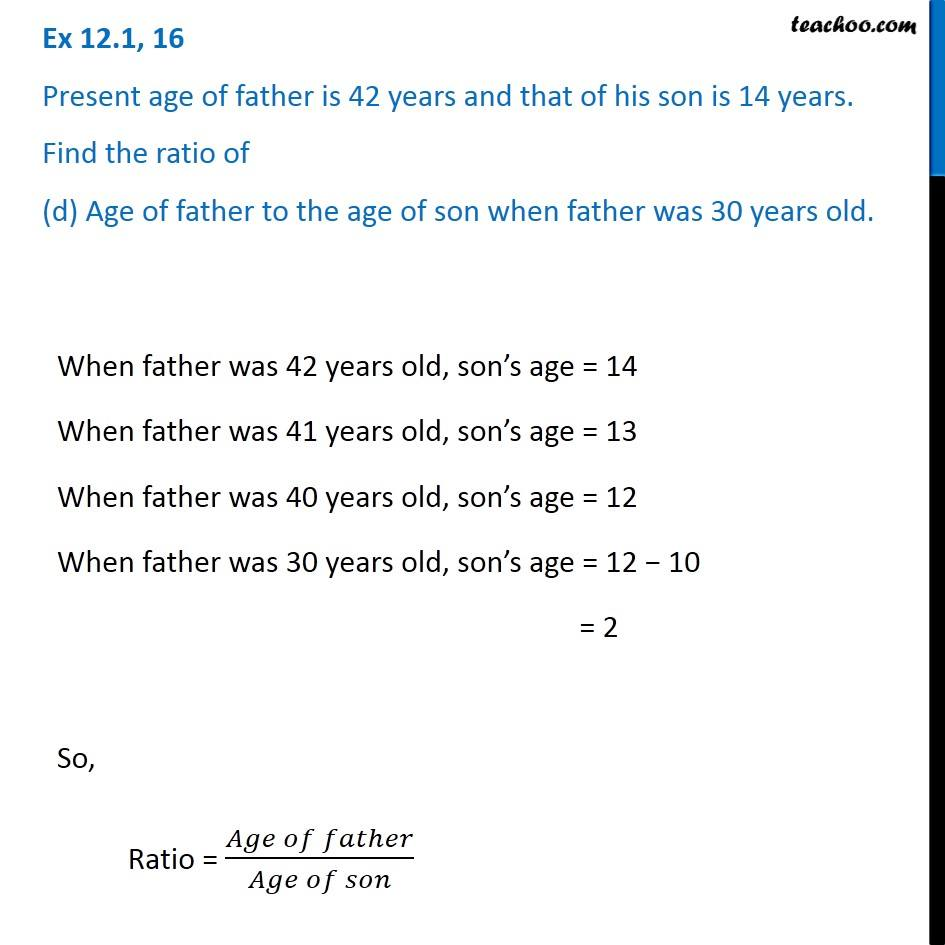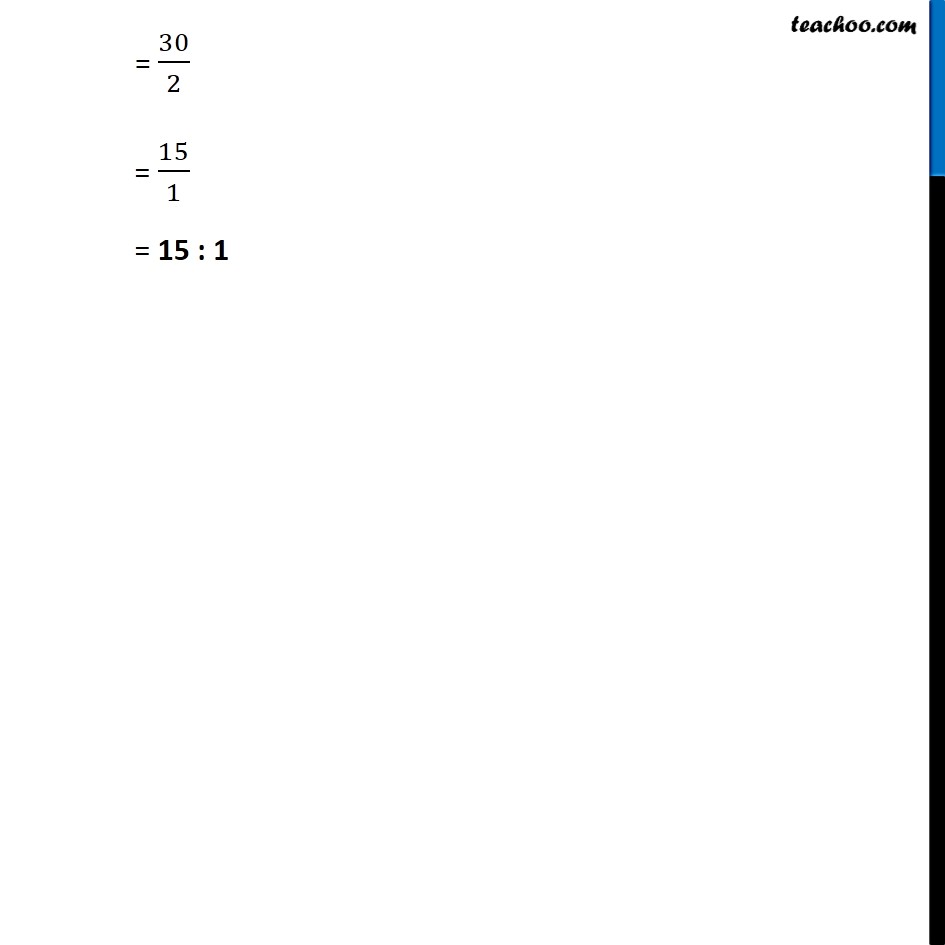Learn in your speed, with individual attention - Teachoo Maths 1-on-1 Class

### Transcript

Ex 12.1, 16 - Chapter 12 Class 6 Ratio and Proportion - NCERT Present age of father is 42 years and that of his son is 14 years. Find the ratio of (a) Present age of father to the present age of son. Present age of father = 42 years Present age of son = 14 years So, Ratio = (𝑃𝑟𝑒𝑠𝑒𝑛𝑡 𝑎𝑔𝑒 𝑜𝑓 𝑓𝑎𝑡ℎ𝑒𝑟)/(𝑃𝑟𝑒𝑠𝑒𝑛𝑡 𝑎𝑔𝑒 𝑜𝑓 𝑠𝑜𝑛) = 42/14 = 21/7 = 3/1 = 3 : 1 Ex 12.1, 16 Present age of father is 42 years and that of his son is 14 years. Find the ratio of (b) Age of the father to the age of son, when son was 12 years old. Age of father when son was 14 years old = 42 Age of father when son was 13 years old = 41 Age of father when son was 12 years old = 40 So, Ratio = (𝐴𝑔𝑒 𝑜𝑓 𝑓𝑎𝑡ℎ𝑒𝑟)/(𝐴𝑔𝑒 𝑜𝑓 𝑆𝑜𝑛) = 40/12 = 20/6 = 10 : 3 Ex 12.1, 16 Present age of father is 42 years and that of his son is 14 years. Find the ratio of (c) Age of father after 10 years to the age of son after 10 years. Age of father after 10 years = Present age of father + 10 = 42 + 10 = 52 years Age of son after 10 years = Present age of son + 10 = 14 + 10 = 24 years So, Ratio = (𝐴𝑔𝑒 𝑜𝑓 𝑓𝑎𝑡ℎ𝑒𝑟 𝑎𝑓𝑡𝑒𝑟 10 𝑦𝑒𝑎𝑟𝑠)/(𝐴𝑔𝑒 𝑜𝑓 𝑠𝑜𝑛 𝑎𝑓𝑡𝑒𝑟 10 𝑦𝑒𝑎𝑟𝑠) = 52/24 = 26/12 = 13/6 = 13 : 6 Ex 12.1, 16 Present age of father is 42 years and that of his son is 14 years. Find the ratio of (d) Age of father to the age of son when father was 30 years old.When father was 42 years old, son’s age = 14 When father was 41 years old, son’s age = 13 When father was 40 years old, son’s age = 12 When father was 30 years old, son’s age = 12 − 10 = 2 So, Ratio = (𝐴𝑔𝑒 𝑜𝑓 𝑓𝑎𝑡ℎ𝑒𝑟)/(𝐴𝑔𝑒 𝑜𝑓 𝑠𝑜𝑛) = 30/2 = 15/1 = 15 : 1